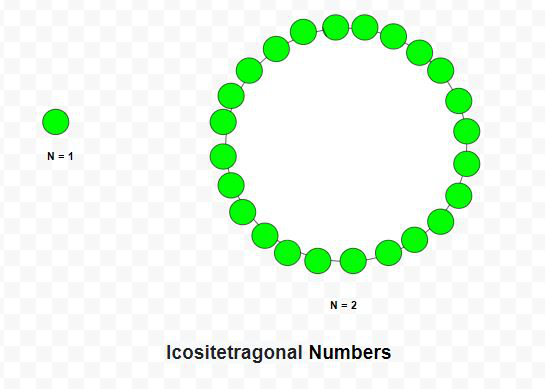# Icositetragonal Number

Given a number N, the task is to find the Nth Icositetragonal number.

An Icositetragonal number is a class of figurate number. It has a 24-sided polygon called Icositetragon. The N-th Icositetragonal number count’s the number of dots and all others dots are surrounding with a common sharing corner and make a pattern.

Examples:

Input: N = 2
Output: 24

Input: N = 6
Output: 336Approach: The Nth icositetragonal number is given by the formula:

``

Below is the implementation of the above approach:

## C++

 `// C++ program to find nth` `// Icositetragonal number`   `#include ` `using` `namespace` `std;`   `// Function to find` `// Icositetragonal number` `int` `Icositetragonal_num(``int` `n)` `{` `    ``// Formula to calculate nth` `    ``// Icositetragonal number` `    ``return` `(22 * n * n - 20 * n) / 2;` `}`   `// Driver Code` `int` `main()` `{` `    ``int` `n = 3;`   `    ``cout << Icositetragonal_num(n) << endl;`   `    ``n = 10;`   `    ``cout << Icositetragonal_num(n);`   `    ``return` `0;` `}`

## Java

 `// Java program to find nth` `// icositetragonal number` `import` `java.util.*;`   `class` `GFG {` `    `  `// Function to find` `// icositetragonal number` `static` `int` `Icositetragonal_num(``int` `n)` `{` `    `  `    ``// Formula to calculate nth` `    ``// icositetragonal number` `    ``return` `(``22` `* n * n - ``20` `* n) / ``2``;` `}`   `// Driver code` `public` `static` `void` `main(String[] args)` `{` `    ``int` `n = ``3``;` `    ``System.out.println(Icositetragonal_num(n));` `    `  `    ``n = ``10``;` `    ``System.out.println(Icositetragonal_num(n));` `}` `}`   `// This code is contributed by offbeat`

## Python3

 `# Python3 program to find nth ` `# Icositetragonal number `   `# Function to find` `# Icositetragonal number` `def` `Icositetragonal_num(n):` `    `  `    ``# Formula to calculate nth ` `    ``# Icositetragonal number ` `    ``return` `(``22` `*` `n ``*` `n ``-` `20` `*` `n) ``/` `2`   `# Driver Code` `n ``=` `3` `print``(``int``(Icositetragonal_num(n))) `   `n ``=` `10` `print``(``int``(Icositetragonal_num(n))) `   `# This code is contributed by divyeshrabadiya07`

## C#

 `// C# program to find nth` `// icositetragonal number` `using` `System;`   `class` `GFG{` `    `  `// Function to find` `// icositetragonal number` `static` `int` `Icositetragonal_num(``int` `n)` `{` `    `  `    ``// Formula to calculate nth` `    ``// icositetragonal number` `    ``return` `(22 * n * n - 20 * n) / 2;` `}`   `// Driver code` `public` `static` `void` `Main(``string``[] args)` `{` `    ``int` `n = 3;` `    ``Console.Write(Icositetragonal_num(n) + ``"\n"``);` `    `  `    ``n = 10;` `    ``Console.Write(Icositetragonal_num(n) + ``"\n"``);` `}` `}`   `// This code is contributed by rutvik_56`

## Javascript

 ``

Output:

```69
1000```

Time Complexity: O(1)

Auxiliary Space: O(1)

Feeling lost in the world of random DSA topics, wasting time without progress? It's time for a change! Join our DSA course, where we'll guide you on an exciting journey to master DSA efficiently and on schedule.
Ready to dive in? Explore our Free Demo Content and join our DSA course, trusted by over 100,000 geeks!

Previous
Next